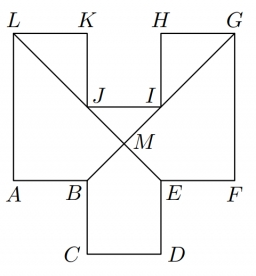In the ABCDEFGHIJKL, the two adjacent sides are perpendicular to each other, and all sides except the AL and GF sides are identical. The AL and GF parties are twice as long as the other parties. The lines BG and EL intersect at point M and divide the dodecagon into six shapes (three triangles, two quadrilaterals, and one pentagon). The EFGM square has an area of 7 cm2.

Determine the area of the other five departments.

S1 =  7 cm2
S2 =  5 cm2
S3 =  2 cm2
S4 =  2 cm2
S5 =  1 cm2

### Step-by-step explanation:Did you find an error or inaccuracy? Feel free to write us. Thank you!

Tips for related online calculators
Need help calculating sum, simplifying, or multiplying fractions? Try our fraction calculator.

#### Grade of the word problem:

We encourage you to watch this tutorial video on this math problem: